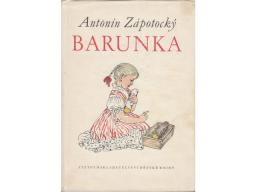# Granddaughter 2789

Grandma and her granddaughter Barunka have a birthday on the same day. During six consecutive birthday celebrations, Grandma's age was always divisible by Barunka's age. How many birthdays did Grandma celebrate at the last of these six celebrations? Grandma is not more than 100 years old.

x =  66

### Step-by-step explanation:

B=61, V=1; B/V=61
B=62, V=2; B/V=31
B=63, V=3; B/V=21
B=64, V=4; B/V=16
B=65, V=5; B/V=13
B=66, V=6; B/V=11Did you find an error or inaccuracy? Feel free to write us. Thank you!

Tips for related online calculators
Do you want to calculate the least common multiple of two or more numbers?
Do you want to calculate the greatest common divisor of two or more numbers?
Do you want to convert time units like minutes to seconds?